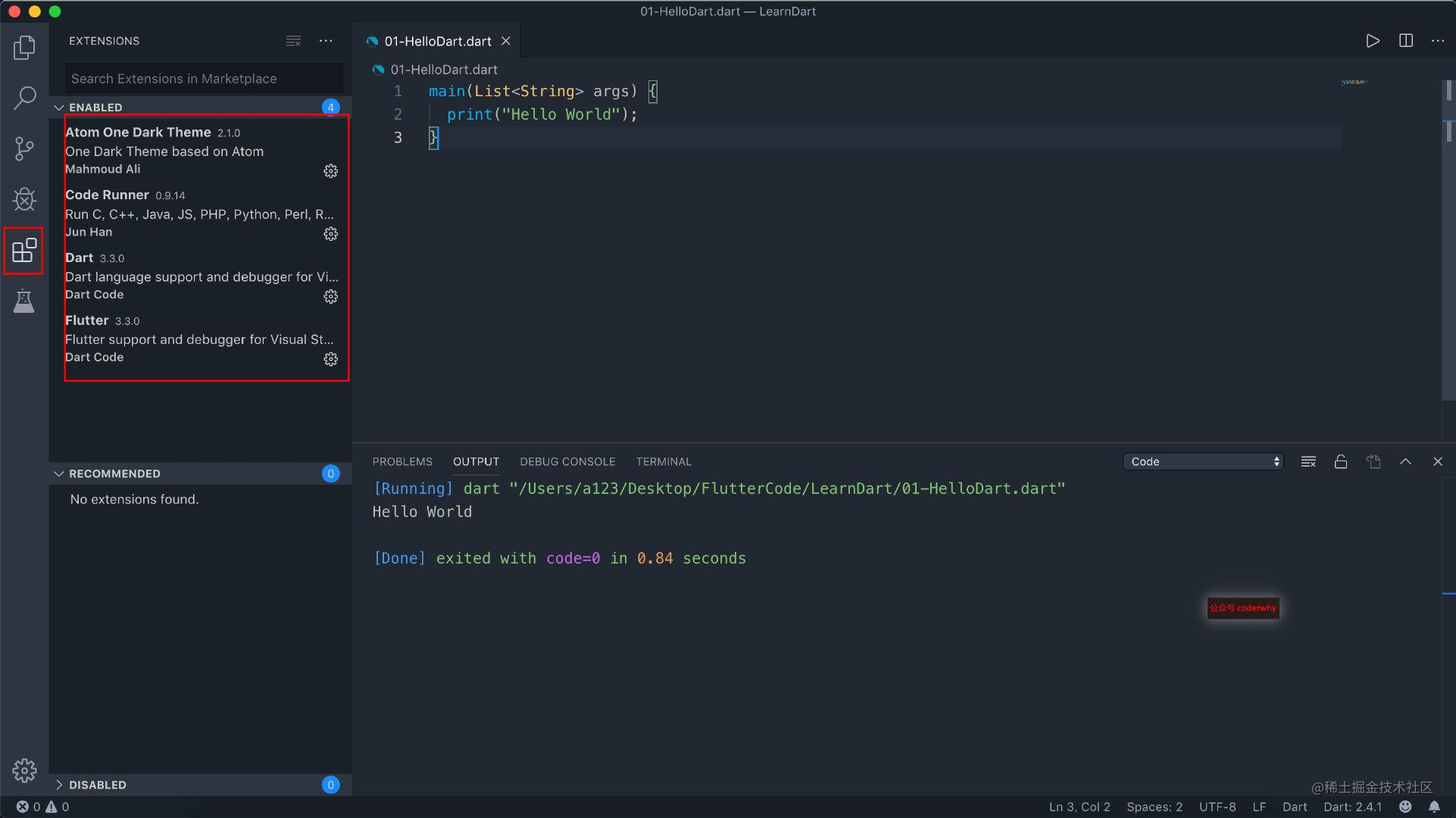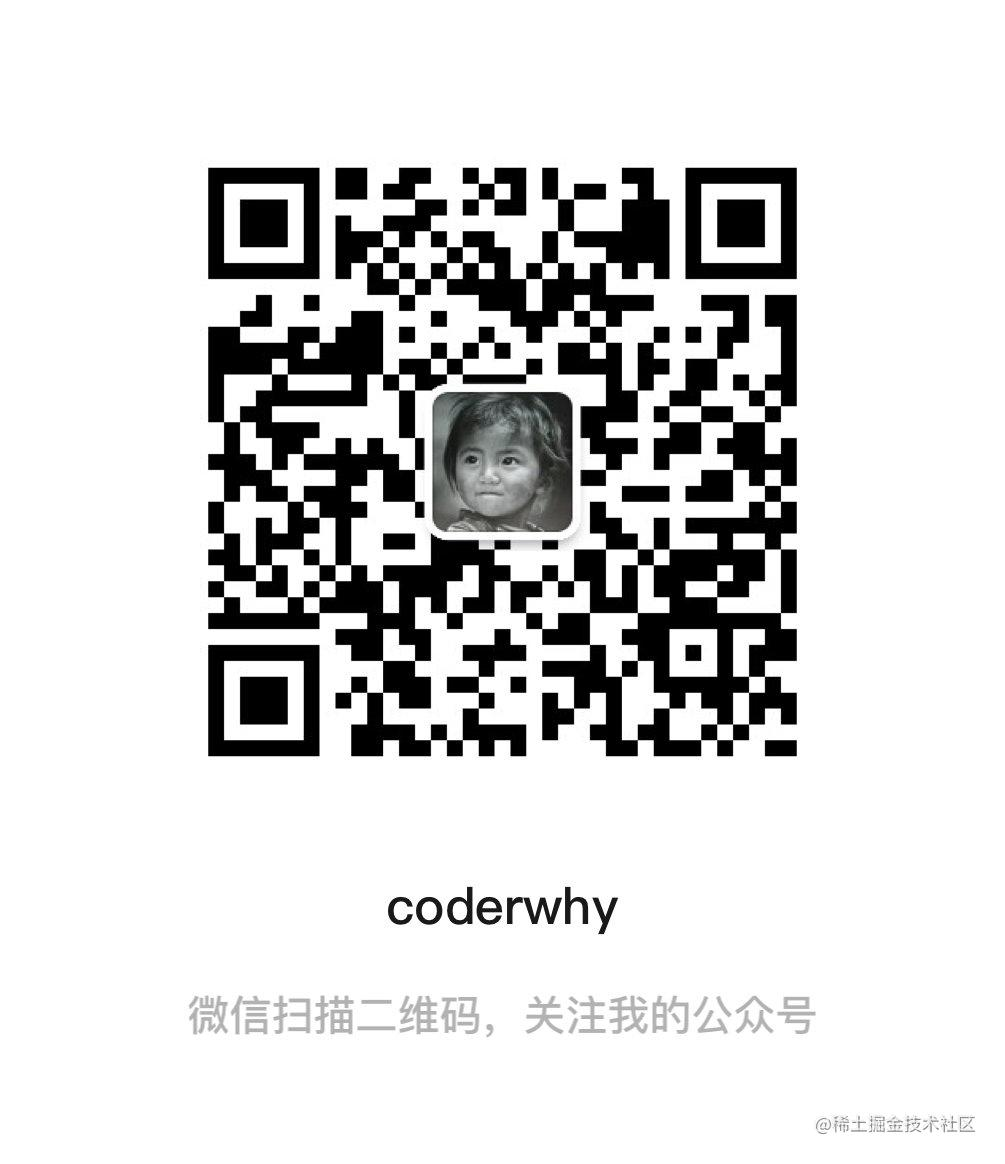# Flutter(三)之搞定Dart（一）

## 一. Dart介绍和安装

### 1.1. 认识Dart

• 因为你学过N种编程语言之后，你会发现他们的`差异是并不大`
• 无非就是`语法上的差异`+`某些语言有某些特性`，而某些语言没有某些特性而已；
• 在我初次接触Flutter的时候，并没有专门去看Dart的语法，而是对于某些语法不太熟练的时候回头去了解而已；

• 我也并不会所有特性都一一罗列，我会挑出比较重要的语言特性来专门讲解；
• 某些特性可能会等到后面讲解Flutter的一些知识的时候单独拿出来讲解；

### 1.2. 安装Dart

1.通过工具安装

• Windows可以通过Chocolatey
• macOS可以通过homebrew
• 具体安装操作官网网站有详细的解释

2.直接下载SDK，配置环境变量

• 下载地址：dart.dev/tools/sdk/a…
• 我采用了这个安装方式。
• 下载完成后，根据路径配置环境变量即可。

### 1.3. VSCode配置

• 一方面编写代码非常方便，而且界面风格我也很喜欢
• 另一方面我可以快速在终端看到我编写代码的效果

• Dart和Flutter插件是为Flutter开发准备的
• Atom One Dark Theme是我个人比较喜欢的一个主题
• Code Runner可以点击右上角的按钮让我快速运行代码## 二. Hello Dart

### 2.1. Hello World

``````main(List<String> args) {
print('Hello World');
}

### 2.2. 程序的分析

• 一、Dart语言的入口也是main函数，并且必须显示的进行定义；
• 二、Dart的入口函数`main`是没有返回值的；
• 三、传递给`main`的命令行参数，是通过`List<String>`完成的。
• 从字面值就可以理解`List`是Dart中的集合类型。
• 其中的每一个`String`都表示传递给`main`的一个参数；
• 四、定义字符串的时候，可以使用单引号或双引号；
• 五、每行语句必须使用分号结尾，很多语言并不需要分号，比如Swift、JavaScript；

## 三. 定义变量

### 3.1. 明确声明(Explicit)

``````变量类型 变量名称 = 赋值;

``````String name = 'coderwhy';
int age = 18;
double height = 1.88;
print('\${name}, \${age}, \${height}'); // 拼接方式后续会讲解

``````String content = 'Hello Dart';
content = 'Hello World'; // 正确的
content = 111; // 错误的, 将一个int值赋值给一个String变量

### 3.2. 类型推导(Type Inference)

``````var/dynamic/const/final 变量名称 = 赋值;

#### 3.3.1. var的使用

var的使用示例:

• runtimeType用于获取变量当前的类型
``````var name = 'coderwhy';
name = 'kobe';
print(name.runtimeType); // String

var的错误用法:

``````var age = 18;
age = 'why'; // 不可以将String赋值给一个int类型

#### 3.3.2. dynamic的使用

• 但是在开发中, 通常情况下不使用dynamic, 因为类型的变量会带来潜在的危险
``````dynamic name = 'coderwhy';
print(name.runtimeType); // String
name = 18;
print(name.runtimeType); // int

#### 3.3.3. final&const的使用

final和const都是用于定义常量的, 也就是定义之后值都不可以修改

``````final name = 'coderwhy';
name = 'kobe'; // 错误做法

const age = 18;
age = 20; // 错误做法

final和const有什么区别呢?

• const在赋值时, 赋值的内容必须是在编译期间就确定下来的
• final在赋值时, 可以动态获取, 比如赋值一个函数
``````String getName() {
return 'coderwhy';
}

main(List<String> args) {
const name = getName(); // 错误的做法, 因为要执行函数才能获取到值
final name = getName(); // 正确的做法
}

final和const小案例:

• 首先, const是不可以赋值为DateTime.now()
• 其次, final一旦被赋值后就有确定的结果, 不会再次赋值
``````// const time = DateTime.now(); // 错误的赋值方式
final time = DateTime.now();
print(time); // 2019-04-05 09:02:54.052626

sleep(Duration(seconds: 2));
print(time); // 2019-04-05 09:02:54.052626

const放在赋值语句的右边，可以共享对象，提高性能:

• 这里可以暂时先做了解，后面讲解类的常量构造函数时，我会再次提到这个概念
``````class Person {
const Person();
}

main(List<String> args) {
final a = const Person();
final b = const Person();
print(identical(a, b)); // true

final m = Person();
final n = Person();
print(identical(m, n)); // false
}

## 四. 数据类型

### 4.1. 数字类型

``````// 1.整数类型int
int age = 18;
int hexAge = 0x12;
print(age);
print(hexAge);

// 2.浮点类型double
double height = 1.88;
print(height);

``````// 字符串和数字转化
// 1.字符串转数字
var one = int.parse('111');
var two = double.parse('12.22');
print('\${one} \${one.runtimeType}'); // 111 int
print('\${two} \${two.runtimeType}'); // 12.22 double

// 2.数字转字符串
var num1 = 123;
var num2 = 123.456;
var num1Str = num1.toString();
var num2Str = num2.toString();
var num2StrD = num2.toStringAsFixed(2); // 保留两位小数
print('\${num1Str} \${num1Str.runtimeType}'); // 123 String
print('\${num2Str} \${num2Str.runtimeType}'); // 123.456 String
print('\${num2StrD} \${num2StrD.runtimeType}'); // 123.46 String

### 4.2. 布尔类型

``````// 布尔类型
var isFlag = true;
print('\$isFlag \${isFlag.runtimeType}');

Dart的类型安全性意味着您不能使用if(非booleanvalue)或assert(非booleanvalue)之类的代码。

``````  var message = 'Hello Dart';
// 错误的写法
if (message) {
print(message)
}

### 4.3. 字符串类型

Dart字符串是UTF-16编码单元的序列。您可以使用单引号或双引号创建一个字符串:

``````// 1.定义字符串的方式
var s1 = 'Hello World';
var s2 = "Hello Dart";
var s3 = 'Hello\'Fullter';
var s4 = "Hello'Fullter";

``````// 2.表示多行字符串的方式
var message1 = '''

``````// 3.拼接其他变量
var name = 'coderwhy';
var age = 18;
var height = 1.88;
print('my name is \${name}, age is \$age, height is \$height');

### 4.4. 集合类型

#### 4.4.1. 集合类型的定义

``````// List定义
// 1.使用类型推导定义
var letters = ['a', 'b', 'c', 'd'];
print('\$letters \${letters.runtimeType}');

// 2.明确指定类型
List<int> numbers = [1, 2, 3, 4];
print('\$numbers \${numbers.runtimeType}');

• 其实，也就是把`[]`换成`{}`就好了。
• `Set``List`最大的两个不同就是：`Set`是无序的，并且元素是不重复的。
``````// Set的定义
// 1.使用类型推导定义
var lettersSet = {'a', 'b', 'c', 'd'};

// 2.明确指定类型
Set<int> numbersSet = {1, 2, 3, 4};

``````// Map的定义
// 1.使用类型推导定义
var infoMap1 = {'name': 'why', 'age': 18};
print('\$infoMap1 \${infoMap1.runtimeType}');

// 2.明确指定类型
Map<String, Object> infoMap2 = {'height': 1.88, 'address': '北京市'};
print('\$infoMap2 \${infoMap2.runtimeType}');

#### 4.4.2. 集合的常见操作

``````// 获取集合的长度
print(letters.length);
print(infoMap1.length);

• 并且，对`List`来说，由于元素是有序的，它还提供了一个删除指定索引位置上元素的方法
``````// 添加/删除/包含元素

numbers.remove(1);

print(numbers.contains(2));

// List根据index删除元素
numbers.removeAt(3);
print('\$numbers');

• 由于它有key和value，因此无论是读取值，还是操作，都要明确是基于key的，还是基于value的，或者是基于key/value对的。
``````// Map的操作
// 1.根据key获取value
print(infoMap1['name']); // why

// 2.获取所有的entries
print('\${infoMap1.entries} \${infoMap1.entries.runtimeType}'); // (MapEntry(name: why), MapEntry(age: 18)) MappedIterable<String, MapEntry<String, Object>>

// 3.获取所有的keys
print('\${infoMap1.keys} \${infoMap1.keys.runtimeType}'); // (name, age) _CompactIterable<String>

// 4.获取所有的values
print('\${infoMap1.values} \${infoMap1.values.runtimeType}'); // (why, 18) _CompactIterable<Object>

// 5.判断是否包含某个key或者value
print('\${infoMap1.containsKey('age')} \${infoMap1.containsValue(18)}'); // true true

// 6.根据key删除元素
infoMap1.remove('age');
print('\${infoMap1}'); // {name: why}

## 五. 函数

### 5.1. 函数的基本定义

Dart是一种真正的面向对象语言，所以即使函数也是对象，所有也有类型, 类型就是Function。

``````返回值 函数的名称(参数列表) {
函数体
return 返回值
}

``````int sum(num num1, num num2) {
return num1 + num2;
}

Effective Dart建议对公共的API, 使用类型注解, 但是如果我们省略掉了类型, 依然是可以正常工作的

``````sum(num1, num2) {
return num1 + num2;
}

• 注意, 这里面只能是一个表达式, 不能是一个语句
``````sum(num1, num2) => num1 + num2;

### 5.2. 函数的参数问题

#### 5.2.1. 可选参数

``````命名可选参数: {param1, param2, ...}

``````// 命名可选参数
printInfo1(String name, {int age, double height}) {
print('name=\$name age=\$age height=\$height');
}

// 调用printInfo1函数
printInfo1('why'); // name=why age=null height=null
printInfo1('why', age: 18); // name=why age=18 height=null
printInfo1('why', age: 18, height: 1.88); // name=why age=18 height=1.88
printInfo1('why', height: 1.88); // name=why age=null height=1.88

``````// 定义位置可选参数
printInfo2(String name, [int age, double height]) {
print('name=\$name age=\$age height=\$height');
}

// 调用printInfo2函数
printInfo2('why'); // name=why age=null height=null
printInfo2('why', 18); // name=why age=18 height=null
printInfo2('why', 18, 1.88); // name=why age=18 height=1.88

``````// 命名可选参数的必须
printInfo3(String name, {int age, double height, @required String address}) {
}

#### 5.2.2. 参数默认值

• 注意, 只有可选参数才可以有默认值, 必须参数不能有默认值
``````// 参数的默认值
printInfo4(String name, {int age = 18, double height=1.88}) {
print('name=\$name age=\$age height=\$height');
}

Dart中的main函数就是一个接受可选的列表参数作为参数的, 所以在使用main函数时, 我们可以传入参数, 也可以不传入

### 5.3. 函数是一等公民

``````main(List<String> args) {
// 1.将函数赋值给一个变量
var bar = foo;
print(bar);

// 2.将函数作为另一个函数的参数
test(foo);

// 3.将函数作为另一个函数的返回值
var func =getFunc();
func('kobe');
}

// 1.定义一个函数
foo(String name) {
print('传入的name:\$name');
}

// 2.将函数作为另外一个函数的参数
test(Function func) {
func('coderwhy');
}

// 3.将函数作为另一个函数的返回值
getFunc() {
return foo;
}

### 5.4. 匿名函数的使用

``````main(List<String> args) {
// 1.定义数组
var movies = ['盗梦空间', '星际穿越', '少年派', '大话西游'];

// 2.使用forEach遍历: 有名字的函数
printElement(item) {
print(item);
}
movies.forEach(printElement);

// 3.使用forEach遍历: 匿名函数
movies.forEach((item) {
print(item);
});
movies.forEach((item) => print(item));
}

### 5.5. 词法作用域

dart中的词法有自己明确的作用域范围，它是根据代码的结构({})来决定作用域范围的

``````var name = 'global';
main(List<String> args) {
// var name = 'main';
void foo() {
// var name = 'foo';
print(name);
}

foo();
}

### 5.6. 词法闭包

``````main(List<String> args) {
return (num i) {
};
}

}

### 5.7. 返回值问题

``````main(List<String> args) {
print(foo()); // null
}

foo() {
print('foo function');
}